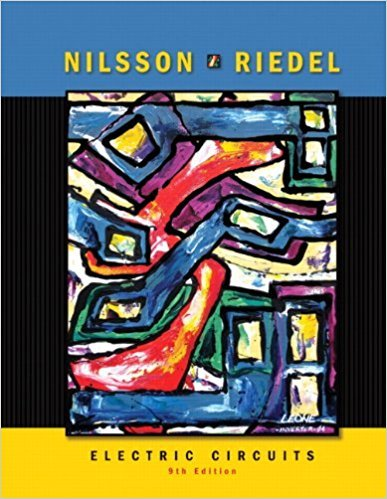×
×

# Solved: The voltage and current at the terminals of the circuit element in Fig 1.5 areISBN: 9780136114994 391

## Solution for problem 1.6 Chapter 1

Electric Circuits | 9th Edition

• Textbook Solutions
• 2901 Step-by-step solutions solved by professors and subject experts
• Get 24/7 help from StudySoup virtual teaching assistantsElectric Circuits | 9th Edition

4 5 1 306 Reviews
10
4
Problem 1.6

The voltage and current at the terminals of the circuit element in Fig 1.5 are zero for t < 0. For f0 , they are v = 80,000f 0; i = 15te-5QQt A, t > 0.a) Find the time when the power delivered to the circuit element is maximum. b) Find the maximum value of power. c) Find the total energy delivered to the circuit element.

Step-by-Step Solution:
Step 1 of 3

l,ACrt (x()= =7f Ynr+ - r- tP) E : Y, + -.: l',Oz pt)T .;h J\. VorCu O ig.^) +N .-\z =- t 14'l, ,ltr 41 /...

Step 2 of 3

Step 3 of 3

##### ISBN: 9780136114994

Electric Circuits was written by and is associated to the ISBN: 9780136114994. This textbook survival guide was created for the textbook: Electric Circuits, edition: 9. Since the solution to 1.6 from 1 chapter was answered, more than 221 students have viewed the full step-by-step answer. The full step-by-step solution to problem: 1.6 from chapter: 1 was answered by , our top Engineering and Tech solution expert on 03/13/18, 07:50PM. This full solution covers the following key subjects: . This expansive textbook survival guide covers 18 chapters, and 1109 solutions. The answer to “The voltage and current at the terminals of the circuit element in Fig 1.5 are zero for t < 0. For f0 , they are v = 80,000f 0; i = 15te-5QQt A, t > 0.a) Find the time when the power delivered to the circuit element is maximum. b) Find the maximum value of power. c) Find the total energy delivered to the circuit element.” is broken down into a number of easy to follow steps, and 66 words.

Unlock Textbook Solution